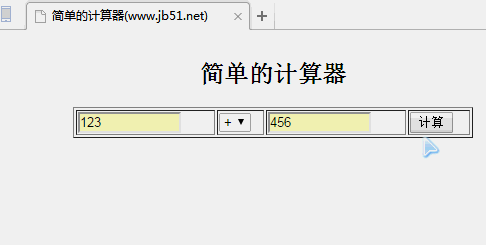PHP实现的简单在线计算器功能示例

```<html>
<meta http-equiv="content-type" content="text/html; charset=utf8">
<title>简单的计算器(www.gimoo.net)</title>
<body>
<?php
//如果用户单击了计算按钮才存在表单变量，才能接收表单数据和对数据进行验证
//以下if语句判断是否将表单中的两个操作数提交到本页面，如果没有则不执行
if(isset(\$_POST["num1"]) && isset(\$_POST["num2"])){
if(empty(\$_POST["num1"])){
//如果第一个第一个操作数为空输出错误信息，并停止计算
echo "<font color='red'>第一个操作数不能为空</font>";
unset(\$_POST["sub"]);
//取消表单中的提交变量，后面计算判断时将不执行
}
if(empty(\$_POST["num2"])){
//如果第二个第一个操作数为空输出错误信息，并停止计算
echo "<font color='red'>第二个操作数不能为空</font>";
unset(\$_POST["sub"]);
//取消表单中的提交变量，后面计算判断时将不执行
}
\$oper=\$_POST["oper"];
//获取操作符号
\$num1=\$_POST["num1"];
//获取第一个操作数
\$num2=\$_POST["num2"];
//获取第二个操作数
if(\$oper == "/"){
if(\$num2 == 0){
echo "<font color='red'>0不能作为除数使用</font>";
unset(\$_POST["sub"]);
}
}
}
?>
<!--以HTML表格的形式输出计算器的用户操作界面-->
<table border="1" align="center" width="400">
<form action="" method="post"> <!--使用POST方法提交给本页面-->
<caption><h2>简单的计算器</h2></caption>
<tr>
<td><input type="text" size="10" name="num1" value="<?php if(!empty(\$num1)){echo \$num1;}?>"></td>
<td>
<select name="oper">
<option value="+" <?php if(\$oper == "+"){echo "selected";}?>>+</option>
<option value="-" <?php if(\$oper == "-"){echo "selected";}?>>-</option>
<option value="*" <?php if(\$oper == "*"){echo "selected";}?>>*</option>
<option value="/" <?php if(\$oper == "/"){echo "selected";}?>>/</option>
</select>
</td>
<td><input type="text" size="10" name="num2" value="<?php if(!empty(\$num2)){echo \$num2;}?>"></td>
<td><input type="submit" name="sub" value="计算"></td>
</tr>
<?php
/**通过表单传过来的\$_POST["sub]，判断是否单击了计算按钮

if(isset(\$_POST["sub"]) && !empty(\$_POST["sub"])){
\$sum = 0; //声明一个存放计算结果的变量，初始值为0
echo \$sum;
//使用switch语句，通过表单选择的操作符来决定执行哪种运算
switch(\$oper){
case "+":
\$sum = \$num1+\$num2;
break;
case "-":
\$sum = \$num1-\$num2;
break;
case "*":
\$sum = \$num1*\$num2;
break;
case "/":
\$sum = \$num1/\$num2;
break;
}
//以下的三行是在表格的新行中输出计算结果
echo "<tr><td colspan='4' align='center'>";
echo "计算结果:\$num1 \$oper \$num2 = \$sum";
echo "</td></tr>";
}
?>
</form>
</table>
</body>
</html>

```PS：这里再为大家推荐几款计算工具供大家进一步参考借鉴：

http://tools.gimoo.net/jisuanqi/equ_jisuanqi

http://tools.gimoo.net/jisuanqi/jsqkexue

http://tools.gimoo.net/jisuanqi/jsq

phpStudy中升级MySQL版本到5.7.17的方法步骤

PHP7扩展开发教程之Hello World实现方法示例